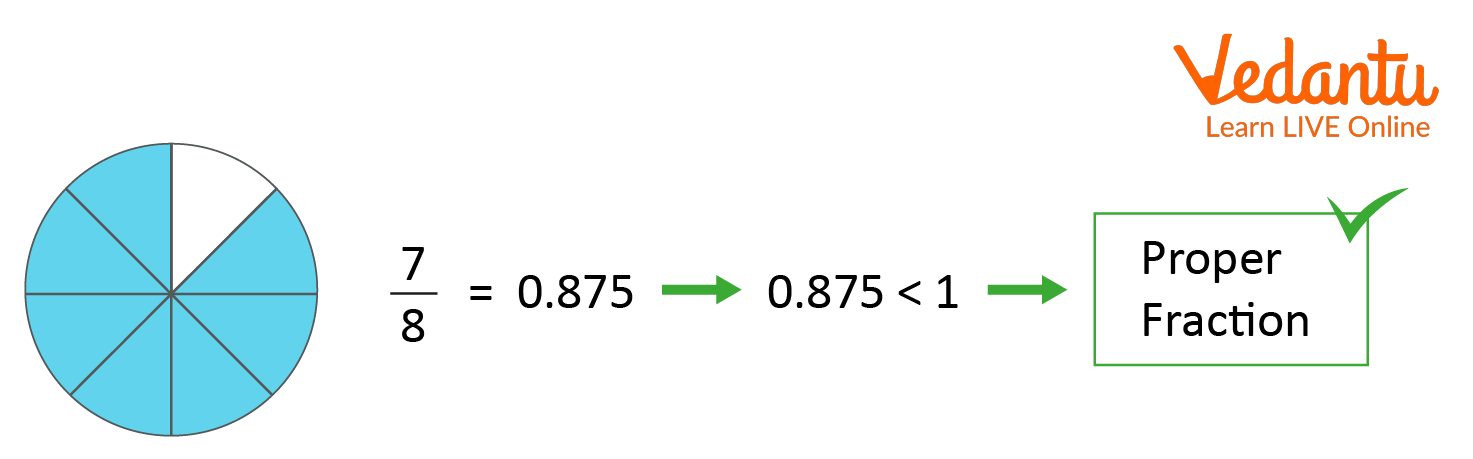Courses
Courses for Kids
Free study material
Offline Centres
More

# Fraction Less than One and Its ExamplesLast updated date: 03rd Dec 2023
Total views: 160.2k
Views today: 1.60k## An Introduction to the Fraction Less Than One

Student often think that all fractions are less than one. But this is wrong, some fractions are less than one, some are greater than one and some are equal to one. A fraction is composed of two parts, one is the numerator and the other is the denominator. A fraction will be less than one means the numerator is lower than the denominator. For example, ⅓ is a fractional number. Here, the numerator is 1 and the denominator is 3 and we can see that 1<3; so, it will be a fraction less than one. This type of fraction is called a proper fraction. It is a type of fraction.

### Examples of Fractions Less Than One

Suppose you have three pizzas. In the first two images, there are four slices of pizza. When two-slice are left, it will one half of the pizza which is ½ and when one-quarter of the pizza is left, then it will be ¼ and in the last image of pizza, there will be eight slices and only three are left, so the fraction part which is left will be ⅜. Hence, through these examples, it is clear that a proper fraction is just a fraction where the numerator is less than the denominator.One HalfOne QuarterThree Eighth

Let’s see one more example for a better understanding. Let's take a fraction where the numerator is lower than the denominator. Suppose the fraction is ⅞. If we will calculate the value of ⅞, it will be equal to 0.875 which is less than one. Hence, it is a fraction whose value is less than one and called a proper fraction.Example of Proper fraction

### Conclusion

Fraction and its types are important and basic concepts of Mathematics. In this article, we have learnt about fractions less than one which is also called proper fractions. The concept of a fraction less than one is clearly explained through examples.

## FAQs on Fraction Less than One and Its Examples

1. What is a fraction less than one?

A fraction less than one means the value of the fraction after solving will be less than one. This type of fraction is termed a proper fraction where the value of the numerator is less than the denominator. For example, 4/7 is a proper fraction because 4 is the numerator and its value is less than the denominator which is 7. The overall value of the fraction is less than one which is 0.571.

2. What are proper and improper fractions?

Proper and improper fractions both are a type of fractions. A proper fraction is defined as a fraction whose numerator is less than its denominator. Examples of the proper fractions are 3/7 and 7/15 because 3 < 7 and 7 < 15. An improper fraction is defined as a fraction whose numerator is greater than or equal to its denominator. For example, 7/5 and 67/7 are improper fractions because 7 > 5 and 67>7.